# Coloring of Chordal Graphs

• Difficulty Level : Easy
• Last Updated : 02 Mar, 2022

In mathematical graph theory, a chordal graph is a closed-loop having 4 or more vertices such that an edge can be drawn from one vertex to another vertex is present in it. In other words, a chordal graph is a graph of length 4 or more containing at least one chord. A chord is nothing but an edge connecting two vertices of the graph and is drawn inside the graph.

Important Points to Remember:

• A chordal graph is a subset of the complete graph.
• A graph containing no chord is called a hole or chordless cycle.
• A complete graph is a chordal graph itself.

1) Consider the below graph ABCD c. An edge CB is drawn from vertex C to vertex B which acts as a chord in this graph. Hence the below graph is said to be a chordal graph.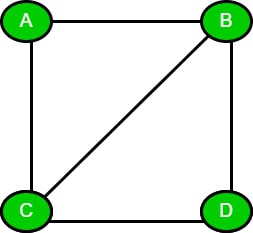Chordal Graph

2) Consider the below example of the complete graph. A graph consisting of n*(n-1)/2 edges is said to be a complete graph, where n is the number of vertices. In the complete graph, the edges connecting all vertices are already present and act as a chord also. Hence, a complete graph is a superset of a chordal graph.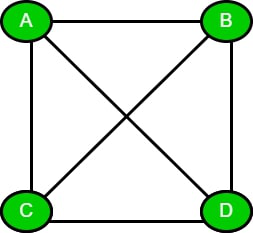Complete graph

### Minimum-coloring Algorithm :

In this article, we will discuss coloring the chordal graphs by using the greedy coloring algorithm.

#### Greedy Coloring Algorithm:

1. First, we need to find the perfect elimination ordering (PEO) of the given chordal graph.
2. Then, traverse the vertices in the reverse order of that PEO that we have already found.
3. Gives the smallest coloring to each vertex such that the current vertex color is not used in its neighboring vertices.
4. If the color of the current vertex is already given to its neighbor then increment the color and assign it to the current vertex.
5. Repeat steps 3 and 4 until all the vertices are colored.

Example: Consider the below chordal graph ABCD. Let the perfect elimination ordering of this graph be [D, C, B, A]. We will traverse the graph PEO in the reverse direction. Hence, [A, B, C, D] is the reverse of PEO.

1. First, we will color the vertex A with 1 as we need to color with the smallest present color.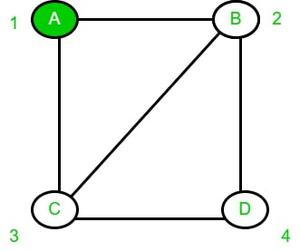2. Now for vertex B, we will check whether its neighbors contain color 1 or not. As vertex ‘A’ contains color 1, therefore we will increment the color by 1 to color vertex B.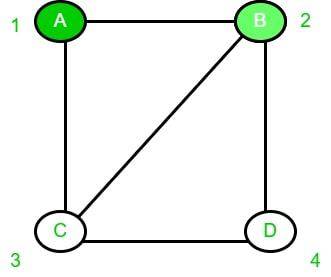3. Similarly, for vertex C, its neighbor A contains color 1, and B contains color 2 so we will color vertex C to 3.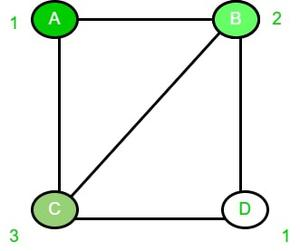4. Now, for vertex D, as its neighbors do not contain the color 1 so we will color 1 to vertex D.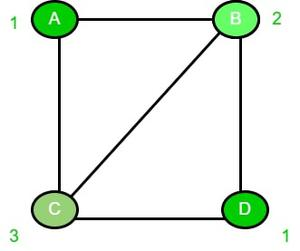My Personal Notes arrow_drop_up